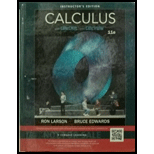Chapter 4.4, Problem 24E### Calculus (MindTap Course List)

11th Edition
Ron Larson + 1 other
ISBN: 9781337275347

#### Solutions

Chapter
Section### Calculus (MindTap Course List)

11th Edition
Ron Larson + 1 other
ISBN: 9781337275347
Textbook Problem

# Evaluating a Definite Integral In Exercises 9-36, evaluate the definite integral. Use a graphing utility to verify your result. ∫ − 8 − 1 x − x 2 2 x 3   d x

To determine

To calculate: The value of the definite integral 81xx22x3dx and to verify it.

Explanation

Given:

The definite integral 81xx22x3dx.

Formula Used:

The following integration formulas hold:

xkdx=xk+1k+1+c

Calculation:

The definite integral can be computed as:

81xx22x3dx=12(81x23dx81x53dx)=12([<

### Still sussing out bartleby?

Check out a sample textbook solution.

See a sample solution

#### The Solution to Your Study Problems

Bartleby provides explanations to thousands of textbook problems written by our experts, many with advanced degrees!

Get Started

## Additional Math Solutions

#### Evaluate the expression sin Exercises 116. 52535252

Finite Mathematics and Applied Calculus (MindTap Course List)

#### Differentiate the function. f(t) = 2t3 3t2 4t

Single Variable Calculus: Early Transcendentals, Volume I

#### Use the following matrices for Problems 1-28. 5. Write the negative of matrix F.

Mathematical Applications for the Management, Life, and Social Sciences

#### True or False: If f(x) 0 for all x in an interval I then f is increasing on I.

Study Guide for Stewart's Single Variable Calculus: Early Transcendentals, 8th